# SET Theory Game

A set is a collection of objects or groups of objects. These objects are often called elements or members of a set. For example, a set of all squares.

A set can be understood playfully using a set game that constitutes 3 components. The three components are

• Colour
• Shape
• Size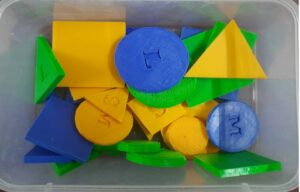It comprises:

Colour: Blue, yellow and green

Shape: Square, triangle, and circle

Size: small, medium, and large.

In total, there are 27 pieces which include

9 triangles (x3 colours, x3 shapes):

The three blue triangles are small, medium, and large.

The three yellow triangles are small, medium, and large.

The three green triangles are small, medium, and large.

9 squares (x3 colours, x3 shapes):

The three small blue squares, medium, and large.

The three small yellow squares, medium, and large.

The three small green squares, medium, and large.

9 circles (x3 colours, x3 shapes):

The three blue circles are small, medium, and large.

The three yellow circles are small, medium, and large.

The three green circles are small, medium, and large.

This set game can be used by children for a better understanding of set theory concepts.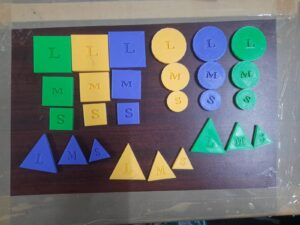Set Operations:

The four important set operations that can be performed using this set game are:

• Union of sets
• Intersection of sets
• Complement of sets
• Difference of sets

Union of sets:

The union of two sets is a set containing all elements that are in A or B (possibly both). The union of sets can be denoted using the symbol ‘U’. Symbolically, we can represent the union of A and B as A U B.

For example, A = Set of blue colour triangles and B = a Set of Green colour triangles.

Then the union will be all the triangles which are in blue and green.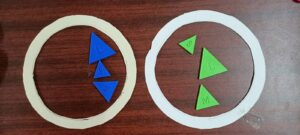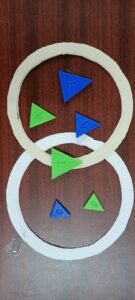The intersection of sets:

The intersection of two sets A and B is the set of all those elements which are common to both A and B. The intersection of sets can be denoted using the symbol ‘∩’. Symbolically, we can represent the intersection of A and B as A ∩ B.

For example, A = Set of blue colour objects and B = a Set of all triangles.

Then the intersection will be the triangles which are in blue.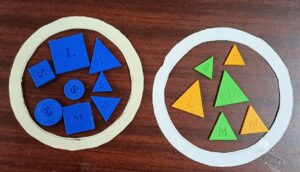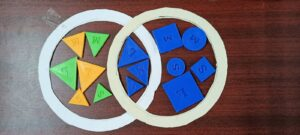Compliment of sets:

The complement of set A is defined as a set that contains the elements present in the universal set but not in set A. The complement of set A can be denoted using the symbol A’.

For example, A = Set of blue colour circles and U = Set of all circles

Then the complement of A, A’ will be circles which are in yellow and green.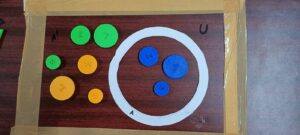Difference of sets:

The difference between sets A and B in this order is the set of elements which belong to A but not to B. Symbolically, we write A – B and read as “ A minus B”.

For example, A = Set of all green colour objects and B = Set of all squares.

Then the difference between A and B, A-B will be set of all green colour objects except squares.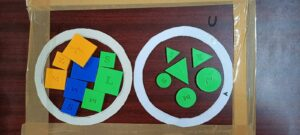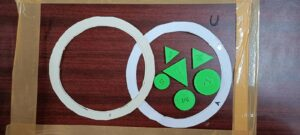Fundamental Properties of Set operations that can be observed:

The operations such as union and intersection in set theory obey the properties of associativity and commutativity. Also, the intersection of sets distributes over the union of sets.

Similarly, the set theory game can be used for understanding three sets and their operations.